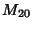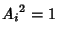## Finite Group Z2Z4One of the three Abelian groups of the five groups total of Order 8. Examples include the Modulo Multiplication Groups,,, and. The elementsof this group satisfy, where 1 is the Identity Element, and four of the elements satisfy. The Cycle Graph is shown above.

See also Finite Group D4, Finite Group Q8, Finite Group Z2Z2Z2, Finite Group Z8